Definitions of Square Dance Calls and Concepts

Index -->  Plus  |  A1  |  A2  |  C1  |  C2  |  C3A  |  C3B  |  C4  |  NOL  |
Definitions (Text Only) -->  Plus  |  A1  |  A2  |  C1  |  C2  |  C3A  |  C3B  |  C4  |  NOL  |
 Find call:

\$BE,@Z\$J(B formation \$B\$+\$i(B.

Sidetrack \$B\$O(B 3 \$B%Q!<%H\$N%3!<%k\$G\$9(B.

Single Sidetrack [C3B]: 1 x 4 Column \$B\$+\$i(B. Zig-Zag; Lockit (\$B\$9\$J\$o\$A(B, 1 x 4 \$B\$G\$N(B Counter Rotate); Roll \$B\$r\$7\$^\$9(B. Single Double Pass Thru \$B\$G=*\$o\$j\$^\$9(B.

Split Sidetrack [C3B]: 2 x 4 \$B\$^\$?\$O(B 1 x 8 \$B\$N(B Column \$B\$+\$i(B. Zig-Zag; Split Counter Rotate 1/4; Roll \$B\$r\$7\$^\$9(B.

Split Split Sidetrack [NOL]: \$BE,@Z\$J(B formation \$B\$+\$i(B. Setup \$B\$r(B 2 \$B2s(B Split \$B\$7(B, \$B\$b\$&(B 1 \$B?M(B (\$B0lHL2=\$5\$l\$?(B Tandem \$B\$N0LCV\$K\$\$\$k?M(B) \$B\$HF0\$-\$^\$9(B. Zig-Zag; Hinge (\$B\$9\$J\$o\$A(B, 1 x 2 \$B\$G\$N(B Counter Rotate); Roll \$B\$r\$7\$^\$9(B.

\$BCm
• Column \$B\$+\$i(B, Single Sidetrack \$B\$G\$O(B 1 x 4 formation \$B\$GF0\$-(B; Split Sidetrack \$B\$G\$O(B 2 x 2 formation \$B\$GF0\$-\$^\$9(B.
• Sidetrack \$B8@MU\$NA0\$K(B Split, Single, Once Removed \$B\$J\$I\$N\$h\$&\$J=\$>~8l\$,\$"\$k>l9g\$r=|\$-(B, Counter Rotate 1/4 \$B\$O>o\$K(B All 8 Counter Rotate 1/4 (\$B\$9\$J\$o\$A(B, '\$BBg\$-\$/(B') \$B\$G\$9(B.
•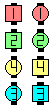\$B\$B;O\$a\$N(B formation: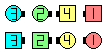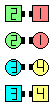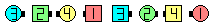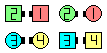Sidetrack\$B\$N8e(B Split Sidetrack\$B\$N8e(B Single Sidetrack\$B\$N8e(B Split Split Sidetrack\$B\$N8e(BChoreography for SidetrackComments? Questions? Suggestions?

https://www.ceder.net/def/sidetrack.php?level=master&language=japan
16-September-2021 04:12:07